# mapprofile

Interpolate between waypoints on terrain

## Syntax

``[zq,distq,latq,lonq] = mapprofile(Z,R,lat,lon)``
``[zq,distq,latq,lonq] = mapprofile(Z,R,lat,lon,units)``
``[zq,distq,latq,lonq] = mapprofile(Z,R,lat,lon,units,trackmethod)``
``[zq,distq,latq,lonq] = mapprofile(Z,R,lat,lon,units,trackmethod,interpmethod)``
``[zq,distq,latq,lonq] = mapprofile(Z,R,lat,lon,ellipsoid)``
``[zq,distq,latq,lonq] = mapprofile(Z,R,lat,lon,ellipsoid,trackmethod)``
``[zq,distq,latq,lonq] = mapprofile(Z,R,lat,lon,ellipsoid,trackmethod,interpmethod)``
``mapprofile(___)``
``[___] = mapprofile``

## Description

### Use Unit Sphere and Get Range in Degrees

````[zq,distq,latq,lonq] = mapprofile(Z,R,lat,lon)` interpolates intermediate points between the waypoints specified by `lat` and `lon`. You must specify the elevation data `Z` and spatial reference `R` for the terrain. For each intermediate point, the function returns the interpolated terrain height in `zq`, the distance from the first waypoint (the range) in `distq`, the latitude in `latq`, and the longitude in `lonq`.```

### Specify Range Units

example

````[zq,distq,latq,lonq] = mapprofile(Z,R,lat,lon,units)` specifies the units `units` for `distq`. The function calculates surface distances using the default radius of the Earth (equivalent to 6371 kilometers).```
````[zq,distq,latq,lonq] = mapprofile(Z,R,lat,lon,units,trackmethod)` additionally specifies whether intermediate points are along great circles or rhumb lines.```

example

````[zq,distq,latq,lonq] = mapprofile(Z,R,lat,lon,units,trackmethod,interpmethod)` additionally specifies the interpolation method.```

### Specify Reference Ellipsoid

````[zq,distq,latq,lonq] = mapprofile(Z,R,lat,lon,ellipsoid)` specifies the reference ellipsoid for the coordinates. This syntax returns `distq` using the units of the semimajor axis of the reference ellipsoid.```
````[zq,distq,latq,lonq] = mapprofile(Z,R,lat,lon,ellipsoid,trackmethod)` additionally specifies whether intermediate points are along great circle tracks or rhumb line tracks.```
````[zq,distq,latq,lonq] = mapprofile(Z,R,lat,lon,ellipsoid,trackmethod,interpmethod)` additionally specifies the interpolation method.```

### Display and Interactively Select Coordinates

````mapprofile(___)` displays an elevation profile of the intermediate points in a new figure on an `axesm`-based map.```

example

````[___] = mapprofile` enables you to interactively select waypoints on the current `axesm`-based map. If the current object on the map is a regular data grid, then the function uses the z-coordinate data (the `ZData` property) as the terrain elevation data. Otherwise, the function uses z-coordinate data from the first regular data grid it finds on the map. If the grid does not have z-coordinate data, then the function uses the color data (the `CData` property), instead. To finish selecting points, press Enter.```

## Examples

collapse all

Load elevation data and a geographic cells reference object for the Korean peninsula. Compute the elevation profile between two points in the region, specifying the units for the range (`distq`) as kilometers. By default, the `mapprofile` function uses bilinear interpolation along a great circle track.

```load korea5c lat = [40.5 30.7]; lon = [121.5 133.5]; [zq,distq,latq,lonq] = mapprofile(korea5c,korea5cR,lat,lon,"km");```

Plot the track over a satellite basemap.

```figure geolimits(korea5cR.LatitudeLimits,korea5cR.LongitudeLimits) hold on geoplot(latq,lonq,"w","LineWidth",2) geobasemap satellite```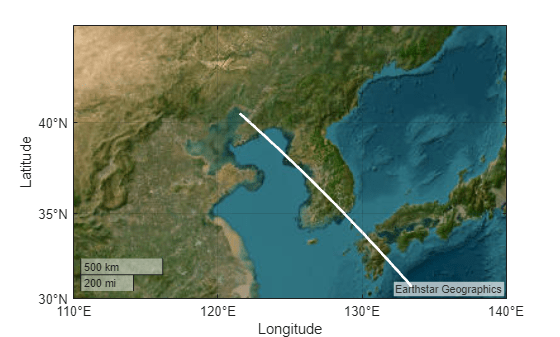Display the elevation profile on a Cartesian plot.

```figure plot(distq,zq) xlabel("Range (kilometers)") ylabel("Elevation (meters)")```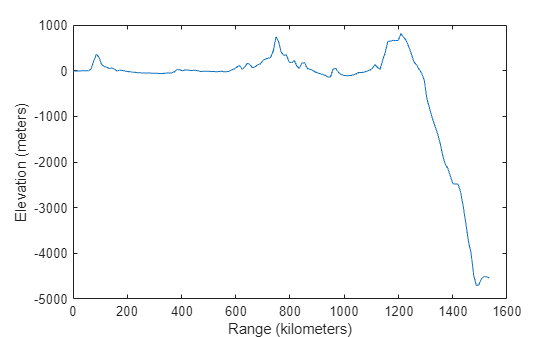Load world topography data into the workspace as an array and a geographic raster reference object. Calculate an elevation profile for the great circle track between San Francisco and New York City, using nearest neighbor interpolation.

```load topo60c lat = [37.77 40.71]; lon = [-122.41 -74.01]; [zqgc,distqgc,latqgc,lonqgc] = mapprofile(topo60c,topo60cR,lat,lon,"km","gc","nearest");```

Calculate an elevation profile for the rhumb line track between the same cities, using bicubic interpolation.

`[zqrh,distqrh,latqrh,lonqrh] = mapprofile(topo60c,topo60cR,lat,lon,"km","rh","bicubic");`

Display both tracks on a map. Use magenta for the great circle track and blue for the rhumb line track.

```figure geoplot(latqgc,lonqgc,"m","LineWidth",1.5) hold on geoplot(latqrh,lonqrh,"b","LineWidth",1.5) geobasemap streets```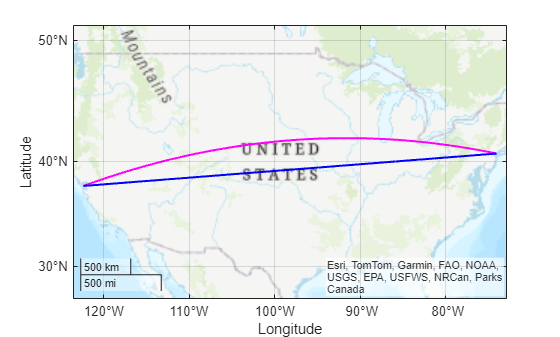Display both elevation profiles on a Cartesian plot.

```figure plot(distqgc,zqgc,"m") hold on plot(distqrh,zqrh,"b") xlabel("Range (kilometers)") ylabel("Elevation (meters)")```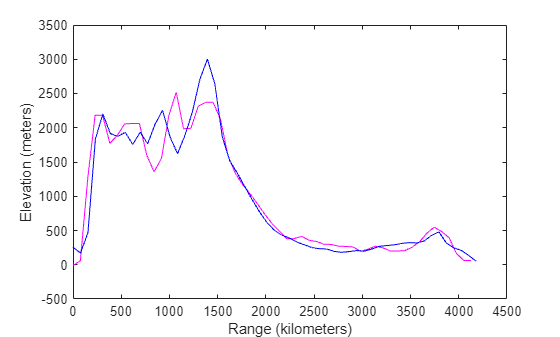Load terrain elevation data for the Korean peninsula into the workspace as an array and a geographic cells reference object. Specify the coordinates of four waypoints.

```load korea5c lat = [43 43 41 38]; lon = [116 120 126 128];```

Display the elevation profile on a map by omitting the output arguments. When you specify more than two waypoints, the function displays the result in 3-D.

`mapprofile(korea5c,korea5cR,lat,lon)`

Add coastlines and city locations to the map.

```load coastlines plotm(coastlat,coastlon) geoshow("worldcities.shp","Marker",".","Color","r")```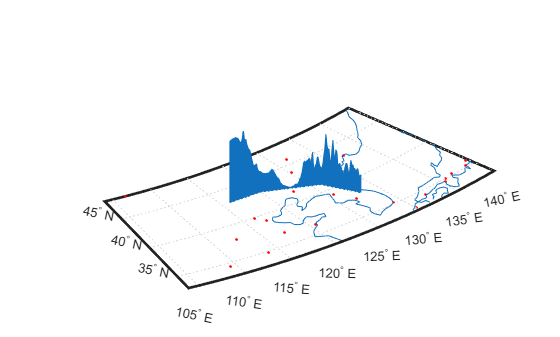## Input Arguments

collapse all

Elevation data grid, specified as an m-by-n array.

Data Types: `single` | `double`

Spatial reference for `Z`, specified as a `GeographicCellsReference` or `GeographicPostingsReference` object. The `RasterSize` property of `R` must be consistent with `size(Z)`.

Latitude coordinates of the waypoints, in degrees, specified as a vector. You can separate sets of waypoints into line sequences using `NaN` values. The `NaN` values in `lat` must correspond to the `NaN` values in `lon`.

The size of `lat` must match the size of `lon`.

Data Types: `single` | `double`

Longitude coordinates of the waypoints, in degrees, specified as a vector. You can separate sets of waypoints into line sequences using `NaN` values. The `NaN` values in `lon` must correspond to the `NaN` values in `lat`.

The size of `lat` must match the size of `lon`.

Data Types: `single` | `double`

Units for `distq`, specified as one of these options:

• These angular units.

ValueUnit Name
`"degree"`, `"degrees"`, `"deg"`Degrees
`"radian"`, `"radians"`, `"rad"`Radians
• Any length unit supported by the `validateLengthUnit` function.

ValueUnit Name
`"m"`, `"meter"`, `"meters"`, `"metre"`, `"metres"`Meters
`"cm"`, `"centimeter"`, `"centimeters"`, `"centimetre"`, `"centimetres"`Centimeters
`"mm"`, `"millimeter"`, `"millimeters"`, `"millimetre"`, `"millimetres"`Millimeters
`"micron"`, `"microns"`Microns
`"km"`,` "kilometer"`, `"kilometers"`, `"kilometre"`, `"kilometers"`Kilometers
`"nm"`, `"naut mi"`, ```"nautical mile"```, `"nautical miles"`Nautical miles
`"ft"`, `"international ft"`, `"foot"`, `"international foot"`, `"feet"`, `"international feet"`Feet
`"in"`, `"inch"`, `"inches"`Inches
`"yd"`, `"yds"`, `"yard"`, `"yards"`Yards
`"mi"`, `"mile"`, `"miles"`, `"international mile"`, `"international miles"`Miles
`"sf"`, `"survey ft"`, ```"US survey ft"```, `"U.S. survey ft"`, ```"survey foot"```, `"US survey foot"`, ```"U.S. survey foot"```, `"survey feet"`, ```"US survey feet"```, `"U.S. survey feet"`U.S. survey feet
`"sm"`, `"survey mile"`, ```"survey miles"```, `"statute mile"`, ```"statute miles"```, `"US survey mile"`, ```"US survey miles"```, `"U.S. survey mile(s)"`, ```"U.S. survey miles"```U.S. survey miles (statute miles)
`"Clarke's foot"`, `"Clarkes foot"`Clarke's feet
`"German legal metre"`, ```"German legal meter"```German legal metres
`"Indian foot"`Indian feet

This argument is case insensitive.

Data Types: `char` | `string`

Reference ellipsoid, specified as a `referenceSphere` object, a `referenceEllipsoid` object, an `oblateSpheroid` object, or a two-element vector of the form `[semimajor_axis eccentricity]`, where `semimajor_axis` is the length of the semimajor axis and `eccentricity` is the eccentricity. The values `semimajor_axis` and `eccentricity` must be of data type `double`.

The default value of `[1 0]` represents the unit sphere.

When you specify the `ellipsoid` argument, the `mapprofile` function returns `distq` in the units of the semimajor axis of the reference ellipsoid.

If you do not specify the `ellipsoid` argument and `R` contains a `geocrs` object in its `GeographicCRS` property, then the `mapprofile` function uses the ellipsoid contained in the `geocrs` object. For example, given a reference object `R`, the function uses `R.GeographicCRS.Spheroid`.

Track method, specified as one of these options:

• `"rh"` — Find track points along rhumb line paths.

• `"gc"` — For spheres, find track points along great circle paths. For ellipsoids, find track points along geodesic paths.

Data Types: `char` | `string`

Interpolation method, specified as one of these options:

• `"bilinear"` — Use linear interpolation.

• `"bicubic"` — Use cubic interpolation.

• `"nearest"` — Use nearest neighbor interpolation.

Data Types: `char` | `string`

## Output Arguments

collapse all

Terrain heights of the intermediate points, returned as a numeric vector. The function calculates the terrain heights using the same units as `Z`.

The number of intermediate points depends on the density of the terrain elevation data. When you increase the density of the data, the `mapprofile` function returns more intermediate points.

The sizes of `zq`, `distq`, `latq`, and `lonq` match.

Range, returned as a numeric vector. For each intermediate point, the range is the distance from the first point to the intermediate point. If you separate the input waypoints into sequences using `NaN` values, then the function calculates the distance of each intermediate waypoint from the first waypoint in the corresponding sequence.

By default, the units for `distq` are degrees. You can specify the units for `distq` using the `units` or `ellipsoid` argument.

The number of intermediate points depends on the density of the terrain elevation data. When you increase the density of the data, the `mapprofile` function returns more intermediate points.

The sizes of `zq`, `distq`, `latq`, and `lonq` match.

Latitudes of the intermediate points, in degrees, returned as a numeric vector.

The number of intermediate points depends on the density of the terrain elevation data. When you increase the density of the data, the `mapprofile` function returns more intermediate points.

The sizes of `zq`, `distq`, `latq`, and `lonq` match.

Longitudes of the intermediate points, in degrees, returned as a numeric vector.

The number of intermediate points depends on the density of the terrain elevation data. When you increase the density of the data, the `mapprofile` function returns more intermediate points.

The sizes of `zq`, `distq`, `latq`, and `lonq` match.

## Version History

Introduced before R2006a

expand all